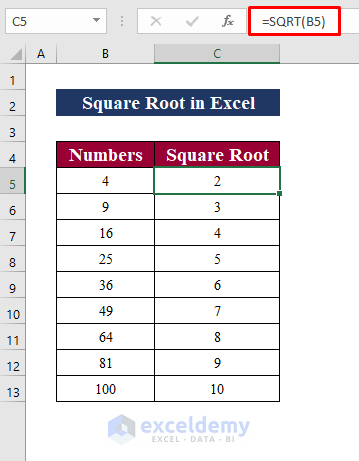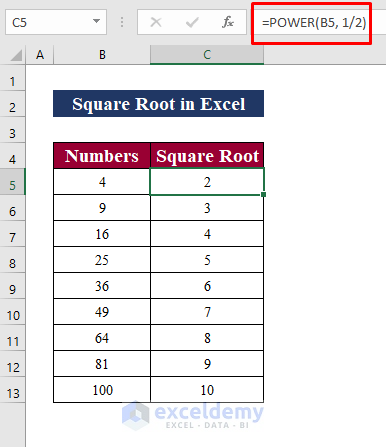# How to Use SQRT function in Excel (6 Suitable Examples)

In Mathematics and Trigonometry, we frequently need to know the square root of a number. The square root of an integer is a value that equals the number when multiplied by itself. MS Excel 2010 was the first to introduce the feature. The image below is a synopsis of the text, showing a few examples of how to use the SQRT function in Excel.In the following sections of this tutorial, you’ll learn more about the methods and other functions that you’ll need to use the SQRT function correctly. In addition to the SQRT function, we’ve discussed some different techniques to find the square root and also the nth root of a number.

## Introduction to the SQRT Function

Function Objective:

The SQRT function in Excel returns the square root of a number.

Syntax:

=SQRT(number)

Arguments Explanation:

Argument Required/Optional Explanation
number Required This is the number we are looking for the square root of. A positive number, an Excel formula, or a function that returns a positive value must be read.

Return Parameter: The Excel SQRT function returns the square root of a positive number like for number 4 it returns the value 2.

## 6 Suitable Examples of Using the SQRT Function in Excel

It’s remarkable how many different methods you can achieve the same thing with Excel. After all, there are a plethora of fantastic features and services. Calculating the square root in Excel is a very easy yet frequently required activity.

And, as I previously stated, there are six suitable ways to accomplish this with Excel (formulas, Power Query).

### 1. Basic Use of SQRT Function in Excel

The simplest way to compute square root in Excel is to use the function that was dedicated specifically for this purpose. The SQRT function returns the square root of a single argument (which could be a number or a reference to a number).

Formula Syntax:

`=SQRT(number)`

Steps:

• At first, type the SQRT function =SQRT(B5)” in cell C5 to calculate the square root of cell C5
• Then, press Enter.

###Therefore, another outcome may be -10, but the formula only yields positive values

### 2. Apply the SQRT Function in Excel for a Negative Number

While this function works well with positive numbers, it will return a #NUM error if you give it a negative number.

This is understandable because a negative number does not have a square root in mathematics. Even if a number is negative, multiplying it by itself produces a positive result.

If you still want to get the square root of a negative number (assuming it was a positive), you’ll need to convert it to a positive number first and then find the square root. You can combine the SQRT function with the ABS function to calculate the square root of -16, -36, -81.

Formula Syntax:

`=SQRT(ABS(number))`

Step 1:

• Firstly, type the SQRT function =SQRT( )
• Type the ABS function= ABS( )” and select cell B5. The formula will appear like that “=SQRT(ABS(B5))
• Press Enter
• Drag down to Auto-fil

### 3. Find Square Root Without the SQRT Function Using the Power Function

The POWER function, unlike the SQRT function, can be used to calculate a number’s roots (such as square root or cube root) or powers (such as square or cube).

The POWER function is essentially another way to do the square root, namely, raise a number to the power of 1/2.

Formula Syntax:

`=POWER(number, power)`

As you might expect, you supply 1/2 to the power argument to get a square root.

Step: 1

##### 4. Get Square Root Using the An Exponent Formula

When calculating manually, the radical symbol (√ ) is used to write the square root. Even though you can’t type the usual square root symbol in Excel, there is a way to find square root without using any functions. The caret character (＾), which is situated above the number 6 on most keyboards, is used for this.

Formula Syntax:

`=number^(power)`

Step 1:

• Select cell B5
• Type Shift+6 to get the caret character (＾)
• Type the power to ½
• Press Enter

### 5. Find Square Root Using the An IF Function

In this following section, we will discuss finding square roots using the IF function. It’s a very important section while working with mixed data containing text values and numbers.

This square root expression can be utilized in larger formulations as well. For example, the IF statement below instructs Excel to generate a square root based on the following condition: If B5 includes a number, return a square root; if B5 is a text value or blank, return a blank cell:

Formula Syntax:

`=IF(ISNUMBER(value), value_if_true,value_if_false)`

Step 1:

• Type the IF function
• Select the logical test to ISNUMBER
• Select value to B5
• Type B5^(1/2) for value_if_true
• Type ” ” (blank value) for value_if_false
• Press EnterTherefore, you will see that it results in square root only for the numbers but blank values for the text values.

### 6. Calculate Nth Root in Excel

Here, there is a bonus section for you! In case you need to calculate up to any root, a similar process to method 3 can be followed to get up to the nth root of a particular number. For example, we want to find the 4th root of the number in cell B13.

Formula Syntax:

`=POWER(number, power)`

Step 1:

Similarly, we can calculate the 4th root of the number in another way like method 4 (Exponent Formula). The exponential operator has the advantage of allowing you to calculate the square root, cube root, or nth root as well.

You can also use it to find the square, cube, or any power of the number.

Formula Text:

`=number^(1/n)`

Step 1:

• just type the desired root in the denominator of a fraction after the caret character (^). Here we type n=4 for the 4th root the numbers in column B.## ✍ Things to Remember

✎ Make sure that fractions (such as 1/2 or 1/3) are enclosed in brackets when applying the exponential operator. For Example, =4^(1/2) and =4^1/2  produce two different outcomes. This is because the exponential operator is calculated first, rather than division. The problem is solved by using brackets.

✎ A #NUM error will be returned if you use a negative number in the POWER function.

## Conclusion

To conclude, I hope this article has given you some useful information about how to apply the SQRT function in Excel. All of these procedures should be learned and applied to your dataset. Take a look at the practice workbook and put these skills to the test. We’re motivated to keep making tutorials like this because of your valuable support.

If you have any questions – Feel free to ask us. Also, feel free to leave comments in the section below.

We, The Exceldemy Team, are always responsive to your queries.

Stay with us & keep learning.

## Related Articles#### Bhubon Costa

Myself, Bhubon Costa, an engineer, is currently employed with Exceldemy as an Excel & VBA Content Developer. I appreciate solving analytical difficulties, finding practical answers, and, for the most part, I enjoy taking on new tasks. I write articles on Exceldemy about various ways to get out of Microsoft Excel's stuck conditions. My passion is to leave my mark on the world through my work and to have an impact on the community who benefit from it.

We will be happy to hear your thoughts